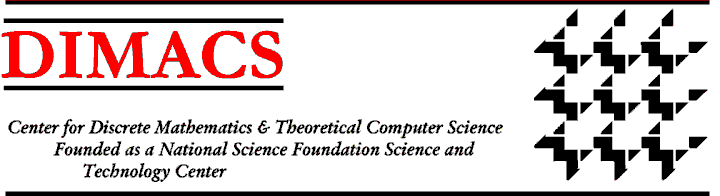### Members of Board of Editors with their scientific interests:

James Abello, DIMACS (abelloj at optonline.net): Discrete and computational geometry and applications; very large graph theory; visualization and animation.

Dick Bumby, Rutgers Math (bumby at math.rutgers.edu): Number theory.

Lisa Carbone, Rutgers Math (carbonel at math.rutgers.edu): Geometric group theory, discrete groups, group actions on trees, combinatorics for problems in algebra, algebra, Kac-Moody groups.

Don Hoover, Rutgers Stat (drhoover at stat.rutgers.edu): Longitudinal methods, infectious disease epidemiology, clustered randomization and multiple comparisons.

Joel Lebowitz, Rutgers Math (lebowitz at math.rutgers.edu): Statistical mechanics of equilibrium and nonequilibrium processes; discrete and continuous time stochastic particle systems; computer simulation of statistical mechanical model systems; foundations of statistical mechanics.

Linda Lesniak, Drew University (llesniak at drew.edu): Combinatorics and graph theory.

Juan Lin, Rutgers Statistics (jklin at stat.rutgers.edu): Multivariate statistics, combinatorics, dimensionality reduction and compression.

Vadim Lozin, Rutgers RUTCOR (lozin at rutcor.rutgers.edu): Special graph classes, subclasses of bipartite graphs are of particular interes; algorithms for hard graph problems on special classes, optimization of stable sets is the problem of extra interest; graph representations and universal graphs.

Avraham Soffer, Rutgers Math (soffer at math.rutgers.edu): Mathematical physics, quantum dynamics of many body systems and environment effects; analysis in probability; limit theorems; nonlinear dynamics and scattering.

Eduardo Sontag, Rutgers Math (sontag at gauss.rutgers.edu): System and control theory; learning and neural networks.

Adriaan van Wijngaarden, Bell Labs (alw at research.bell-labs.com): Information theory, communication theory, combinatorics, networks, coding, modulation, synchronization.

Peter Winkler, Dartmouth (peter.winkler at dartmouth.edu): Discrete math; probability; theory of computing.VolumesDIMACS Homepage
Contacting the Center